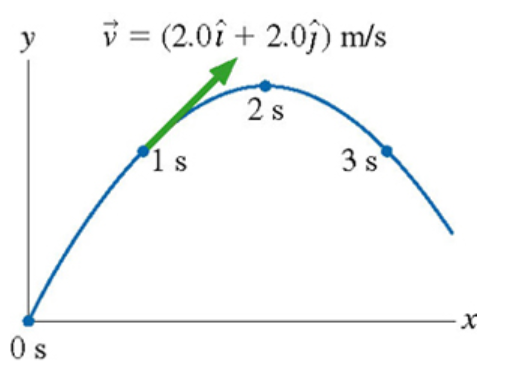# Problem: A physics student on Planet Exidor throws a ball, and it follows the parabolic trajectory shown in the figure. The ball's position is shown at 1s intervals until t=3s. At t=1s , the ball's velocity is v = (2.0 î + 2.0 ĵ) m/s.Determine the ball's velocity at t = 0s.

###### FREE Expert Solution

Uniform accelerated motion (UAM) equations:

We'll use the first UAM equation to solve for the acceleration of the ball:

Using t = 1s and t = 2s, vy changes from 2m/s to 0 m/s

vf,y = v0,y - gt

83% (468 ratings)###### Problem Details

A physics student on Planet Exidor throws a ball, and it follows the parabolic trajectory shown in the figure. The ball's position is shown at 1s intervals until t=3s. At t=1s , the ball's velocity is v = (2.0 + 2.0 ) m/s.

Determine the ball's velocity at t = 0s.